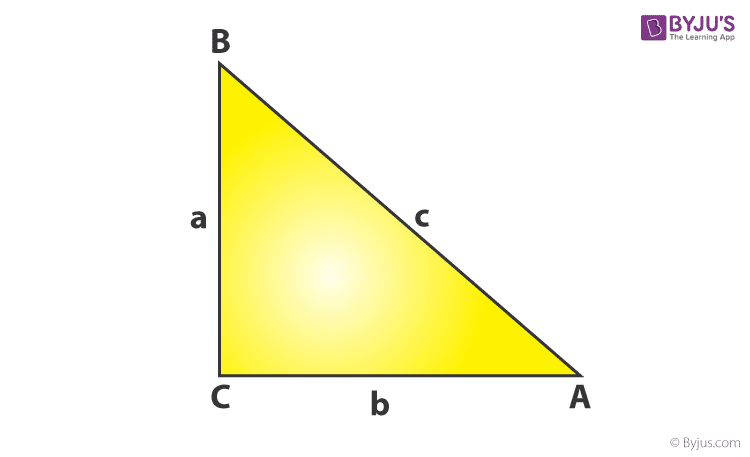# ABC is an isosceles right triangle, right angled at C. Prove that AB2 = 2AC2

ABC is an isosceles right triangle, right angled at C. Prove that AB2 = 2AC2.

Solution:In triangle △ABC, by Pythagorus theorem,

(AB)2 =(AC)2 +(BC)2 …..(1)

Since, △ABC is an isosceles triangle,

AC = BC….(2)

∴ From (1) and (2),

(AB)2 =2(AC)2